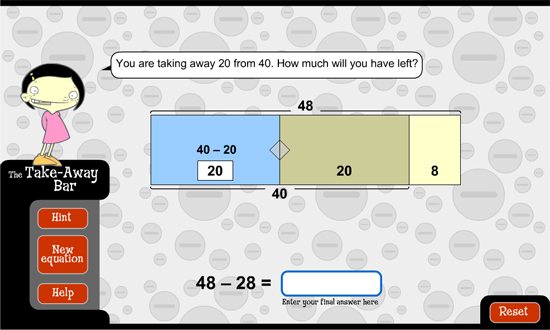﻿ The take-away bar: go figure: subtract ones then tensSubtract ones then tens

This strategy is very similar to 'subtract tens then ones'. This time, take away the ones first. Then, take away the tens.

For example, let's look at 48 minus 28. First, take away 8 (the ones) from 48 to leave 40. Then, take away the 20 (the tens) from 40 to leave 20.Let's find the answer to 57 minus 33 using this strategy. First, take away the ones from 57. Then, take away the tens from that.